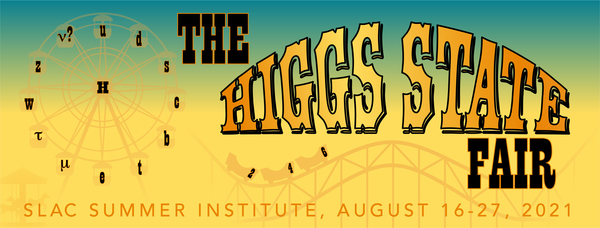#49th SLAC Summer Institute (SSI 2021)

Aug 16 – 27, 2021
SLAC
America/Los_Angeles timezone

## Nonstandard MSSM scenarios with a mass of CP-odd Higgs boson lighter than the top-quark mass

Not scheduled
20m
ZOOM videoconf (SLAC)

### Speaker

Elena Fedotova (SINP MSU)

### Description

The possibility of a mass of a CP-odd Higgs boson, which is lighter than the top quark mass Mt, is considered within the MSSM framework where an effective theory at the scale Mt is the Two-Higgs-doublet model (THDM).

The theoretical analysis was performed in the model approximation where only the effects of the third generation squark mixing are taken into account whenever the other supersymmetric particles decouple, i.e. the scale hierarchy is m_A < Mt < Ms (m_A is a mass of pseudoscalar, Ms is an average mass of stop quarks masses). In this simple approximation, the free parameters are the ratio of Higgs doublet vacuum expectation values tanb, the trilinear couplings of interactions of Higgs fields and third generation of squarks At, Ab, the mass parameter of Higgsino mu, and Ms. Calculation of radiative corrections to the Higgs sector were performed by EFT methods.

It was found that such a possibility is realized in the nonstandard MSSM regime with large At, Ab, and mu (of about 3 Ms), Ms of about 1-2 TeV, tanb of about 2-5, and in a case when 1-loop threshold corrections to dimension-six operators to effective Higgs potential are taken into account.
The analysis of such MSSM regime includes unitarity constraints modified by dimension-six operators, the renormalization scale dependence of 1-loop threshold corrections to self-coupling of Higgs potential (lambda_i, i=1,...7) as well as the RG effects. It was noted that the results are sensitive to the value of the strong coupling constant.

The results were applied to the description of excess at the invariant mass of muon pair 28 GeV with obtained cross-sections of about 0.01–0.40 fb and 0.03–0.90 fb for 8 и 13 TeV correspondingly.

### Primary author

Elena Fedotova (SINP MSU)